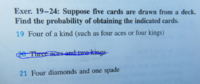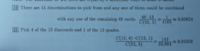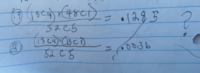# why doesn't this work?

#### allegansveritatem

##### Full Member
Here are the two problems I am going to ask about:Here are the solutions of these two problems from the solutions manual:and here is what I did with them:Now, my question is: Why doesn't my solution for 19 not work? It seems to me that we are choosing 4 cards from 13, no? So why in 19 does the manual say the numerator is 13 times 48 instead of 13C4 times 48? What the soluitons manual seems to be saying is: 13C1 times 48C1....what is the difference in these two problems that the first term in the numerator is different in 19 for the first term in 21?

#### Subhotosh Khan

##### Super Moderator
Staff member
Here are the two problems I am going to ask about:
View attachment 25296

Here are the solutions of these two problems from the solutions manual:
View attachment 25297

and here is what I did with them:
View attachment 25298
Now, my question is: Why doesn't my solution for 19 not work? It seems to me that we are choosing 4 cards from 13, no? So why in 19 does the manual say the numerator is 13 times 48 instead of 13C4 times 48? What the soluitons manual seems to be saying is: 13C1 times 48C1....what is the difference in these two problems that the first term in the numerator is different in 19 for the first term in 21?
Since we had to choose 4 kings or 4 '8 s' etc. - we stack those together.

We would have 13 such stacks.

Now we choose any of those 13 stacks AND any of those rest of 48.

#### HallsofIvy

##### Elite Member
Here's how I would do this question: First, in a standard deck of cards, there are 13 different "kinds" and 4 of each "kind". The first card dealt to you is some "kind". There are then 51 cards left of which 3 cards are that same "kind" so the probability the second card dealt is also of that "kind" is 3/51. There are then 50 cards left of which 2 cards are of that same "kind" so the probability the third card deal is also of that same "kind" is 2/50= 1/25. There are then 49 card left of which 1 card is of that same "kind" so the probability the fourth card is of that same "kind" is 2/49. Finally there are no cards of that same "kind" so the fifth card dealt can be anything.

The probability four cards of the same "kind" are dealt and then a different card is (3/51)(2/50)(1/49)= (3*2*1)/(51*50*49). We can write 3*2*1 as 3!. We can write 1/(51*50*49) as (48*47*...*3*2*1)/(51*50*49*...*3*2*1)= 48!/51! so (3*2*1)/(51*50*49)= 3!(48!)/51!.

Now that is the probability that the four cards of the same "kind" are all dealt first, then the "odd" card. There are, in fact, 4 possible orders- "KKKKO", "KKKOK", "KKOKK", "KOKKK", and "OKKKK". It is easy to show that they all have that same probability so the probability of "four of a kind" is 4(3!)(48!)/51!.

As to why you got a wrong answer, please tell us exactly what you did and why you did it!

#### Jomo

##### Elite Member
Here are the two problems I am going to ask about:
View attachment 25296

Here are the solutions of these two problems from the solutions manual:
View attachment 25297

and here is what I did with them:
View attachment 25298
Now, my question is: Why doesn't my solution for 19 not work? It seems to me that we are choosing 4 cards from 13, no? So why in 19 does the manual say the numerator is 13 times 48 instead of 13C4 times 48? What the soluitons manual seems to be saying is: 13C1 times 48C1....what is the difference in these two problems that the first term in the numerator is different in 19 for the first term in 21?
13C4 is the number of ways you choose 4 objects from 13. In this case it means the number of ways to choose 4 denominations out of 13. Is that really what you want or are you choosing 1 denomination from 13?

#### allegansveritatem

##### Full Member
Since we had to choose 4 kings or 4 '8 s' etc. - we stack those together.

We would have 13 such stacks.

Now we choose any of those 13 stacks AND any of those rest of 48.
Oh! I see it! How dumb I am to have missed that! Yes, each denomination has 4 components! Thanks

#### allegansveritatem

##### Full Member
Here's how I would do this question: First, in a standard deck of cards, there are 13 different "kinds" and 4 of each "kind". The first card dealt to you is some "kind". There are then 51 cards left of which 3 cards are that same "kind" so the probability the second card dealt is also of that "kind" is 3/51. There are then 50 cards left of which 2 cards are of that same "kind" so the probability the third card deal is also of that same "kind" is 2/50= 1/25. There are then 49 card left of which 1 card is of that same "kind" so the probability the fourth card is of that same "kind" is 2/49. Finally there are no cards of that same "kind" so the fifth card dealt can be anything.

The probability four cards of the same "kind" are dealt and then a different card is (3/51)(2/50)(1/49)= (3*2*1)/(51*50*49). We can write 3*2*1 as 3!. We can write 1/(51*50*49) as (48*47*...*3*2*1)/(51*50*49*...*3*2*1)= 48!/51! so (3*2*1)/(51*50*49)= 3!(48!)/51!.

Now that is the probability that the four cards of the same "kind" are all dealt first, then the "odd" card. There are, in fact, 4 possible orders- "KKKKO", "KKKOK", "KKOKK", "KOKKK", and "OKKKK". It is easy to show that they all have that same probability so the probability of "four of a kind" is 4(3!)(48!)/51!.

As to why you got a wrong answer, please tell us exactly what you did and why you did it!
Yes, I see it now. As to what I was thinking....actually I just didn't have a clear picture of what was involved. It was a matter of not thinking it through--an old story. Thanks for your thorough exposition here.

#### HallsofIvy

##### Elite Member
There are 52 cards in a deck and 4 of each of 13 "kinds". The first card could be anything- probability 1. There are then 52 cards in the deck of which 3 are the same "kind" as the first. The probability the second card is that "kind" is 3/51. There are then 50 cards in the deck of which two are the same "kind". The probability the third card is the same "kind" is 2/50. There are then 49 cards in the deck of which 1 is the same "kind". The probability the fourth card is the same "kind" is 1/49. Of course the fifth card is necessarily different so the probability or "KKKKO", where "K" is a card of that "kind" and "O" is the "odd" card is $$\displaystyle 1(3/51)(2/50)(1/49)=$$$$\displaystyle \frac{3(2)(1)}{51(50)(49)}=$$$$\displaystyle \frac{3!(48)(47)\cdot\cdot\cdot(3)(2)(1)}{51(50)(49)(48)(47)\cdot\cdot\cdot (3)(2)(1)}=\frac{3! 48!}{51!}$$. The probability of "KKKOK", "KKOKK", "KOKKK" and "OKKKK" are each the same so the probability of four of a kind in any order is $$\displaystyle \frac{5(3!)(48!)}{51!}$$.

#### Jomo

##### Elite Member
There are 52 cards in a deck and 4 of each of 13 "kinds". The first card could be anything- probability 1. There are then 52 cards in the deck of which 3 are the same "kind" as the first. The probability the second card is that "kind" is 3/51. There are then 50 cards in the deck of which two are the same "kind". The probability the third card is the same "kind" is 2/50. There are then 49 cards in the deck of which 1 is the same "kind". The probability the fourth card is the same "kind" is 1/49. Of course the fifth card is necessarily different so the probability or "KKKKO", where "K" is a card of that "kind" and "O" is the "odd" card is $$\displaystyle 1(3/51)(2/50)(1/49)=$$$$\displaystyle \frac{3(2)(1)}{51(50)(49)}=$$$$\displaystyle \frac{3!(48)(47)\cdot\cdot\cdot(3)(2)(1)}{51(50)(49)(48)(47)\cdot\cdot\cdot (3)(2)(1)}=\frac{3! 48!}{51!}$$. The probability of "KKKOK", "KKOKK", "KOKKK" and "OKKKK" are each the same so the probability of four of a kind in any order is $$\displaystyle \frac{5(3!)(48!)}{51!}$$.
There are 51 cards in the deck of which 3 are the same "kind" as the first.

#### Jomo

##### Elite Member
Here is my take on this.

You want to pick 2 suits from the 13 suits. Then from one of the suits you want to pick 4 out of the 4 available. Then from the other suit you want to pick 1 out of the 4. Then you multiply by 2 as the two suits can be switched wrt how you use them.

13C2*4C4*4C1*2!/52C5

#### Jomo

##### Elite Member
Alternatively...

The 4 of a kind can happen in exactly 13 ways (4-aces or 4-2s or 4-3s or ... or 4 kings). Then you need to pick 1 card from the remaining 48 cards which can happen in exactly 48 ways. So the total number of 4 of a kind poker hands is 13*48. Now divide this by 52C5 and your done.

Edit: SK beat me to this method via post #2

•Subhotosh Khan